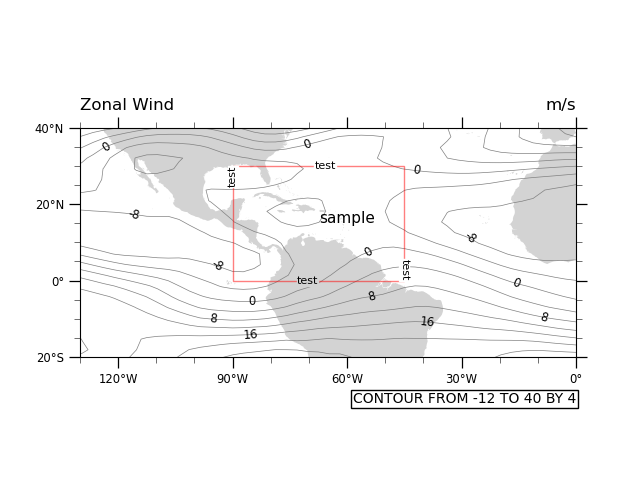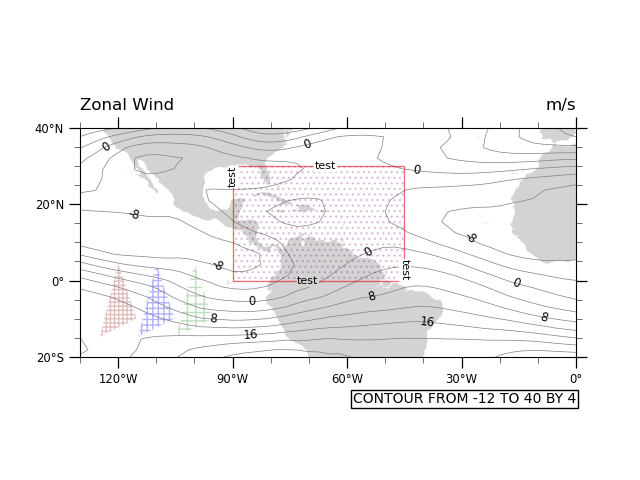# NCL_polyg_4.py#

This script illustrates the following concepts:
• Drawing a cylindrical equidistant map

• Zooming in on a particular area on a cylindrical equidistant map

• Attaching an outlined box to a map plot

• Attaching filled polygons to a map plot

• Filling in polygons with a shaded pattern

• Changing the color and thickness of polylines

• Changing the color of a filled polygon

• Labeling the lines in a polyline

• Changing the density of a fill pattern

• Adding text to a plot

See following URLs to see the reproduced NCL plot & script:

Import packages:

```import numpy as np
import xarray as xr
import cartopy
import cartopy.crs as ccrs
import matplotlib.pyplot as plt

import geocat.datafiles as gdf
import geocat.viz as gv
```

```# Open a netCDF data file using xarray default engine and load the data into xarrays, choosing the 2nd timestamp
ds = xr.open_dataset(gdf.get("netcdf_files/uv300.nc")).isel(time=1)
```

## Utility Function: Make Base Plot:#

```# Define a utility function to create the basic contour plot, which will get used twice to create two slightly
# different plots
def make_base_plot():

# Generate axes using Cartopy projection
ax = plt.axes(projection=ccrs.PlateCarree())

continents = cartopy.feature.NaturalEarthFeature(
name="coastline",
category="physical",
scale="50m",
edgecolor="None",
facecolor="lightgray",
)

# Set map extent
ax.set_extent([-130, 0, -20, 40], crs=ccrs.PlateCarree())

# Define the contour levels. The top range value of 44 is not included in the levels.
levels = np.arange(-12, 44, 4)

# Using a dictionary prevents repeating the same keyword arguments twice for the contours.
kwargs = dict(
levels=levels,  # contour levels specified outside this function
xticks=[-120, -90, -60, -30, 0],  # nice x ticks
yticks=[-20, 0, 20, 40],  # nice y ticks
transform=ccrs.PlateCarree(),  # ds projection
add_labels=False,  # turn off xarray's automatic Lat, lon labels
colors="gray",  # note plurals in this and following kwargs
linestyles="-",
linewidths=0.5,
)

# Contourf-plot data (for filled contours)
hdl = ds.U.plot.contour(x="lon", y="lat", ax=ax, **kwargs)

# Add contour labels.   Default contour labels are sparsely placed, so we specify label locations manually.
# Label locations only need to be approximate; the nearest contour will be selected.
label_locations = [
(-123, 35),
(-116, 17),
(-94, 4),
(-85, -6),
(-95, -10),
(-85, -15),
(-70, 35),
(-42, 28),
(-54, 7),
(-53, -5),
(-39, -11),
(-28, 11),
(-16, -1),
(-8, -9),  # Python allows trailing list separators.
]
ax.clabel(
hdl,
np.arange(-8, 24, 8),  # Only label these contour levels: [-8, 0, 8, 16]
fontsize="small",
colors="black",
fmt="%.0f",  # Turn off decimal points
manual=label_locations,  # Manual label locations
inline=False)  # Don't remove the contour line where labels are located.

# Create a rectangle patch, to color the border of the rectangle a different color.
# Specify the rectangle as a corner point with width and height, to help place border text more easily.
left, width = -90, 45
bottom, height = 0, 30
right = left + width
top = bottom + height

# Draw rectangle patch on the plot
p = plt.Rectangle(
(left, bottom),
width,
height,
fill=False,
zorder=3,  # Plot on top of the purple box border.
edgecolor='red',
alpha=0.5)  # Lower color intensity.

# Draw text labels around the box.
# Change the default padding around a text box to zero, making it a "tight" box.
# Create "text_args" to keep from repeating code when drawing text.
text_shared_args = dict(
fontsize=8,
facecolor='white',
edgecolor='white'),
)

# Draw top text
ax.text(left + 0.6 * width,
top,
'test',
horizontalalignment='right',
verticalalignment='center',
**text_shared_args)

# Draw bottom text.   Change text background to match the map.
ax.text(
left + 0.5 * width,
bottom,
'test',
horizontalalignment='right',
verticalalignment='center',
fontsize=8,
facecolor='lightgrey',
edgecolor='lightgrey'),
)

# Draw left text
ax.text(left,
top,
'test',
horizontalalignment='center',
verticalalignment='top',
rotation=90,
**text_shared_args)

# Draw right text
ax.text(right,
bottom,
'test',
horizontalalignment='center',
verticalalignment='bottom',
rotation=-90,
**text_shared_args)

# Add lower text box.  Box appears off-center, but this is to leave room
# for lower-case letters that drop lower.
ax.text(1.0,
-0.20,
"CONTOUR FROM -12 TO 40 BY 4",
fontname='Helvetica',
horizontalalignment='right',
transform=ax.transAxes,
facecolor='white',
edgecolor='black'))

# Use geocat.viz.util convenience function to add main title as well as titles to left and right of the plot axes.
gv.set_titles_and_labels(ax,
lefttitle="Zonal Wind",
lefttitlefontsize=12,
righttitle="m/s",
righttitlefontsize=12)

# Use geocat.viz.util convenience function to add minor and major tick lines

# Use geocat.viz.util convenience function to make plots look like NCL plots by using latitude, longitude tick labels

return ax
```

## Plot 1 (Text inside a box):#

```# Create the base figure
ax = make_base_plot()

# Draw text inside of box
ax.text(-60.0, 15.0, "sample", fontsize=11, horizontalalignment='center')

# Show the plot
plt.show()
```## Utility Function: Draw Hatch Polygon:#

```# Define a utility function that draws a polygon and then erases its border with another polygon.
def draw_hatch_polygon(xvals, yvals, hatchcolor, hatchpattern):
"""Draw a polygon filled with a hatch pattern, but with no edges on the
polygon."""
ax.fill(
xvals,
yvals,
edgecolor=hatchcolor,
zorder=
-1,  # Place underneath contour map (larger zorder is closer to viewer).
fill=False,
linewidth=0.5,
hatch=hatchpattern,
alpha=0.3  # Reduce color intensity
)

# Hatch color and polygon edge color are tied together, so we have to draw a white polygon edge
# on top of the original polygon to remove the edge.
ax.fill(
xvals,
yvals,
edgecolor='white',
zorder=
0,  # Place on top of other polygon (larger zorder is closer to viewer).
fill=False,
linewidth=1  # Slightly larger linewidth removes ghost edges.
)
```

## Plot 2 (Polygons with hatch patterns):#

```# Make this figure the thumbnail image on the HTML page.
# sphinx_gallery_thumbnail_number = 2

# Create the base figure
ax = make_base_plot()

# Plot the hatch pattern "underneath" the red box, to hide the purple border that is unavoidably attached to producing
# the hatch pattern.
x_points = [-90.0, -45.0, -45.0, -90.0, -90.0]
y_points = [30.0, 30.0, 0.0, 0.0, 30.0]

ax.fill(
x_points,
y_points,
edgecolor='purple',  # Box hatch pattern is purple.
zorder=2,  # Place on top of map (larger zorder is closer to viewer).
fill=False,
hatch='...',  # Adding more or fewer dots to '...' will change hatch density.
linewidth=0.5,  # Make each dot smaller
alpha=0.2  # Make hatch semi-transparent using alpha level in range [0, 1].
)

# Draw some triangles with various hatch pattern densities.
x_tri = np.array([-125, -115, -120])
y_tri = np.array([-15, -10, 5])

draw_hatch_polygon(x_tri, y_tri, 'brown', '++++')

draw_hatch_polygon(x_tri + 10, y_tri, 'blue', '+++')

draw_hatch_polygon(x_tri + 20, y_tri, 'forestgreen', '++')

# Show the plot
plt.show()
```Total running time of the script: ( 0 minutes 0.501 seconds)

Gallery generated by Sphinx-Gallery